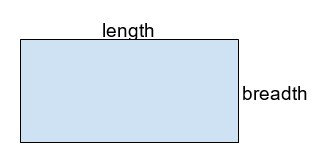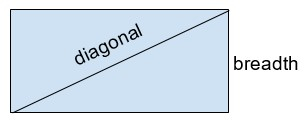# Golang program to find the area of a rectangle

This tutorial will discuss how to find the area of a rectangle in Golang programming using two methods −

• Area of the rectangle using length and breadth

• Area of the rectangle using diagonal and breadth

## Rectangle

A rectangle is a two-dimensional shape that has four sides. The opposite sides of a rectangle are equal and all the angles of a rectangle are at 90°. Another property of a rectangle is that its opposite sides are parallel to each other.

### Area of a rectangle

The total space enclosed within the boundary of the rectangle is known as the area of a rectangle.Its area can be calculated by multiplying the length and breadth of the rectangle.

## Finding the area of a rectangle using length and breadth

Given length ‘l’ and breadth ‘b’ of a rectangle, we need to find out the area of a rectangle.

### Formula

$$\mathrm{Area \, =\, Length * Breadth}$$

### Example

• Length= 5

Area= 5 * 2 = 10

Since, area is calculated by multiplying length and breadth, therefore we multiply 5 and 2, and obtain the area as 10.

• Length= 25.5

Area= 127.5

Area is calculated by multiplying length and breadth, therefore −

Area= 25.5 * 5.0

= 127.5

### Algorithm

Step 1 − Declare two variables- one for storing length ‘l’ and the other one for storing breadth ‘b’ of the rectangle.

Step 2 − Declare a variable for storing the area of the rectangle- ‘area’.

Step 3 − Calculate the area by multiplying the length ‘l’ and breadth ‘b’ of the rectangle and store it in the ‘area’ variable.

Step 4 − Print the calculated area, i.e, the value stored in the variable ‘area’.

## Using integer variables

### Example

package main

// fmt package allows us to print formatted strings
import "fmt"
// function to calculate area of the rectangle using int variable
func calculateAreaOfRectangle(l, b int) {

// integer type variable ‘area’ to store area of the rectangle
var area int

// calculating area by multiplying length ‘l’ and breadth ‘b’
// of the rectangle
area = l * b
fmt.Println("Length ‘l’ =",l)

// printing area of the rectangle
fmt.Println("Therefore, Area of Rectangle : ", area)
}

// driver function
func main() {

// declaring variables for storing length ‘l’ and breadth ‘b’
var l, b = 3, 4

// calling the calculateAreaOfRectangle function for
// calculating the area of the rectangle and passing values
// for length and breadth as parameters
calculateAreaOfRectangle(l, b)
}


### Output

Length ‘l’ = 3
Therefore, Area of Rectangle :  12


### Explanation

In the above code, area is calculated by multiplying the length and breadth of the rectangle using this formula −

area = l * b

Therefore, area= 3 * 4

area= 12

## Using float variables

### Example

package main

// fmt package allows us to print formatted strings
import "fmt"

// function to calculate the area of the rectangle using float variable
func calculateAreaOfRectangle(l, b float32) {

// float type variable ‘area’ to store area of the rectangle
// float32 variable stores smaller value
// float64 variable stores larger value
var area float32

// calculating area by multiplying length ‘l’ and breadth ‘b’
// of the rectangle
area = l * b
fmt.Println("Length ‘l’ =",l)

// printing area of the rectangle
fmt.Println("Therefore, Area of Rectangle : ", area)
}

// driver function
func main() {

// declaring variables for storing length ‘l’ and breadth ‘b’
var l, b float32 = 5.5, 8.5

// calling the calculateAreaOfRectangle function for
// calculating the area of the rectangle and passing values
// for length and breadth as parameters
calculateAreaOfRectangle(l, b)
}


### Output

Length ‘l’ = 5.5
Therefore, Area of Rectangle :  46.75


area= 5.5 * 8.5

= 46.75

## Finding the area of a rectangle using diagonal and breadth

A rectangle has two diagonals and both are equal in size. The area of a rectangle can be calculated using diagonal and breadth.### Formula

$$\mathrm{Area = Breadth \ast (\sqrt{((Diagonal)^{2} - (Breadth)^{2}))}}$$

### Example

• Diagonal= 30.0

Area= 282.842712474619 For calculating the area we are using the values of diagonal and breadth in this formula-

Therefore, Area = 10 * (√((30)2 - (10)2))

Area = 282.842712474619

### Example

package main

// fmt package allows us to print formatted strings
// math package allows us to perform various mathematical
// operations
import (
"fmt"
"math"
)

// function to calculate area of rectangle using float variables
func calculateAreaOfRectangle(d, b float64) {

// float64 type variable ‘area’ to store area of rectangle
var area float64

// calculating area of the rectangle
// math.Sqrt function calculates square root
// math.Pow function calculates the power
fmt.Println("Diagonal ‘d’ =", d)

// calculating area
area = b * math.Sqrt((math.Pow(d, 2) - math.Pow(b, 2)))

// printing area of the rectangle
fmt.Println("Therefore, Area of Rectangle : ", area)
}

// driver function
func main() {

// declaring variables for storing diagonal ‘d’ and breadth ‘b’
var d, b float64 = 40.0, 20.0

// calling the calculateAreaOfRectangle function for
// calculating the area of the rectangle and passing values
// for diagonal and breadth as parameters
calculateAreaOfRectangle(d, b)
}


### Output

Diagonal ‘d’ = 40
Therefore, Area of Rectangle :  692.820323027551


### Explanation

For calculating the area we are using the values of diagonal and breadth in this formula −